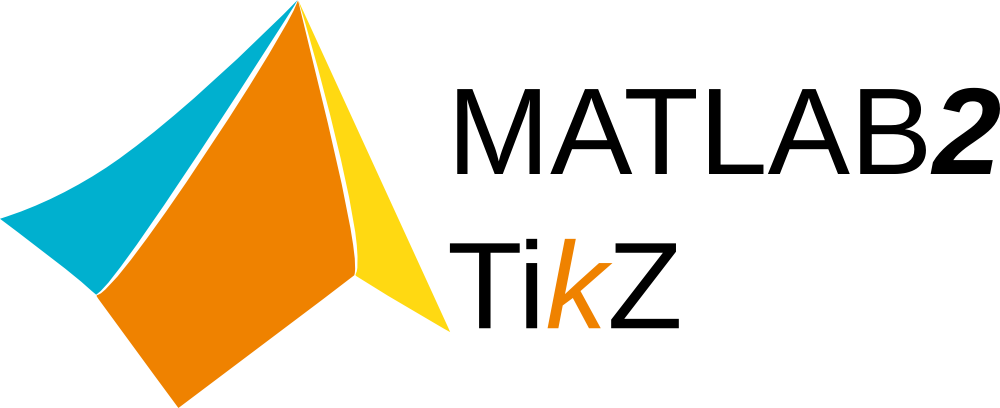# Matlab2tikz

This program converts MATLAB®/Octave figures to TikZ/pgfplots figures for smooth integration into LaTeX.

The updater in matlab2tikz 0.6.0 (and older) no longer works. Please update manually if you are not using matlab2tikz 1.0.0 or newer!matlab2tikz is a MATLAB(R) script to convert native MATLAB(R) figures to TikZ/Pgfplots figures that integrate seamlessly in LaTeX documents.

To download the official releases and rate matlab2tikz, please visit its page on FileExchange.

matlab2tikz converts most MATLAB(R) figures, including 2D and 3D plots. For plots constructed with third-party packages, however, your mileage may vary.

# Installation

1. Extract the ZIP file (or clone the git repository) somewhere you can easily reach it.
2. Add the src/ folder to your path in MATLAB/Octave: e.g.
• by running the addpath function from your command window or startup script.

Make sure that your LaTeX installation is up-to-date and includes:

It is recommended to use the latest stable version of these packages. Older versions may work depending on the actual MATLAB(R) figure you are converting.

# Usage

Typical usage of matlab2tikz consists of converting your MATLAB plot to a TikZ/LaTeX file and then running a LaTeX compiler to produce your document.

## MATLAB

1. Generate your plot in MATLAB(R).

2. Run matlab2tikz, e.g. using

matlab2tikz('myfile.tex');


## LaTeX

Add the contents of myfile.tex into your LaTeX source code, for example using \input{myfile.tex}. Make sure that the required packages (such as pgfplots) are loaded in the preamble of your document as in the example:

\documentclass{article}

\usepackage{pgfplots}
%% the following commands are needed for some matlab2tikz features
\usetikzlibrary{plotmarks}
\usetikzlibrary{arrows.meta}
\usepgfplotslibrary{patchplots}
\usepackage{grffile}
\usepackage{amsmath}

%% you may also want the following commands
%\pgfplotsset{plot coordinates/math parser=false}
%\newlength\figureheight
%\newlength\figurewidth

\begin{document}
\input{myfile.tex}
\end{document}


## Remarks

Most functions accept numerous options; you can check them out by inspecting their help:

help matlab2tikz


Sometimes, MATLAB(R) plots contain some features that impede conversion to LaTeX; e.g. points that are far outside of the actual bounding box. You can invoke the cleanfigure function to remove such unwanted entities before calling matlab2tikz:

cleanfigure;
matlab2tikz('myfile.tex');


• For more information about matlab2tikz, have a look at our GitHub repository. If you are a good MATLAB(R) programmer or LaTeX writer, you are always welcome to help improving matlab2tikz!
• Some common problems and pit-falls are documented in our wiki.
• If you experience (other) bugs or would like to request a feature, please visit our issue tracker.
Open Source Agenda is not affiliated with "Matlab2tikz" Project. README Source: matlab2tikz/matlab2tikz
Stars
1,325
Open Issues
236
Last Commit
3 months ago
Repository